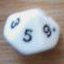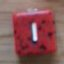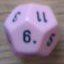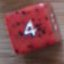Exploding dice

## Exploding dice

 Sat Oct 27 19:54:29 2012   by   Encarmine This is really a great utility.I'm trying to make a set up that deals with exploding dice.The specific is: exploding 2d6 but where every roll of a 6 counts as a 5 and adds another die to be rolled.How would I write this? The presets for exploding 2D6 is otherwise very similar to what I want:sum { sum (accumulate x:=d6 while x=6),      sum (accumulate y:=d6 while y=6) } Mon Oct 29 12:00:01 2012   by   Torben The easiest way to achieve this is to roll normal exploding d6s and then, before adding them up, replacing all 6s by 5s.So something like:`sum 2#(foreach x in accumulate x:=d6 while x=6        do if x=6 then 5 else x)` Mon Oct 29 12:01:49 2012   by   Torben Or, even easier:`sum 2#(foreach x in accumulate x:=d6 while x=6        do min {x,5})`